# Tag Archives: ellipses

[This is the 4th and last installment in the current series on mini-golf and ellipse geometry. See the previous ones here: #1, #2, #3.]

We must settle one more question to round out our elliptical arc: Why does light, when shot from one focus of an ellipse-shaped mirrored room, reflect back to the other focus? To answer this question, we’ll need a Fact, a Formalism, and a Fairy Tale.

### The Fact

Recall that, in the previous post, we saw that ellipses can be described by distances: Any ellipse has two focus points F1 and F2 so that the total length of broken path F1XF2 is the same for every point X on the ellipse; let d be this common total distance. In fact, more is true: the length of F1XF2 is smaller than d if X is inside the ellipse, and larger than d if X is outside.Left: The distance of the broken path is greater, equal, or less than d depending on whether the central vertex is outside, on, or inside the ellipse. Right: A proof of the “outside” case.

To prove this fairly intuitive fact, we’ll use the “straight line principle”: the shortest distance between two points is a straight line. Indeed, when X is outside the ellipse (see right diagram above), straight-line path YF2 is shorter than the path YXF2 that detours through X, and so d = F_1 Y F_2 < F_1 Y X F_2[/latex]. See if you can fill in the case where X is inside the ellipse.

### The Formalism

Recall that when light bounces off a straight mirror, the angle of incidence equals the angle of reflection. But here we’re discussing light bouncing off an ellipse, which is decidedly not straight. So we need to formally describe how light reflects off curved mirrors.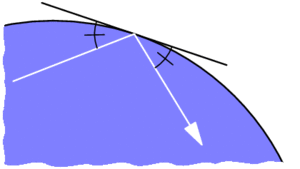Reflection off of a curved mirror behaves like reflection off of the tangent line.

If we zoom into where the incoming light ray strikes a curved mirror (illustrated above), the mirror closely resembles a straight line, specifically its tangent line. This suggests that the light should behave as if it is reflecting off of this line, with equal angles as marked. This is indeed the rule governing ideal reflections on curved mirrors: the angles of incidence and reflection, as measured from the tangent line, should be equal.

### The Fairy Tale

The last ingredient involves Little Red Riding Hood and her thirsty grandmother. Red is delivering cake and wine from her mother (point M) to her grandmother (point G), but she must first fill a bucket of water at the nearby stream S, which is conveniently shaped like a straight line. She was warned by her Brothers to watch out for a big bad wolf, so she must minimize her total walking distance. Where on the stream should she fill her bucket to minimize this distance?Left: Paths from M to S to G transform into paths from M’ to G, the shortest of which is straight. Right: This straight path behaves like a mirror.

To answer this, imagine reflecting the first leg of Red’s journey across line S, so her path from M to S to G gets reflected to a path from M’ to G. The reverse may be done as well: any path from M’ to G turns into a path from M to G that stops somewhere along S. So we just need to find the shortest path from M’ to G. But this is easy: it’s just the straight path M’G. So Red’s shortest path from M to S to G is the one that stops at Z.

Notice that this shortest path is the one with equal angles as marked. This means Red’s best strategy is to pretend the stream is a mirror and to follow the light ray that bounces directly to grandma’s house. This neatly exemplifies Fermat’s principle, which says that light tends to follow the fastest routes.

### The Proof

With these pieces in place, we can finish today’s question in a flash. Let’s say light from focus F1 hits an ellipse at point X, as illustrated below. Why does this ray bounce off the ellipse toward F2? If we draw the tangent line L at point X, by The Formalism above, this question is equivalent to: why does the light ray bounce off of line L toward F2?Why are the two marked angles equal? Because the white path solves Red’s Fairy Tale problem.

Let’s reimagine this Grimm scenario by thinking of F1 as Red’s mother’s house, F2 as grandma’s house, and L as the stream. I claim F1XF2 is the shortest path for Red to take. Why? If Y is any other point on line L, then Y is outside the ellipse, so by The Fact above, F1YF2 has distance longer than d. So F1XF2 is indeed the shortest. But by The Fairy Tale, we know that this shortest route behaves like light bouncing off of line L, i.e., the marked angles are indeed equal. So we’re done!

# What Makes Ellipses… Ellipses?

Last time we used wild properties of ellipses to build some really easy—and some really devilish—golf courses. Specifically, I claimed that every ellipse has two magical points F1 and F2 (called foci) such that a ray from F1 always bounces off the ellipse and lands precisely at F2, and furthermore, this path always has the same length. Why does this happen? And how do we find these foci?

Let’s focus(!) on the last question first. Recall that an ellipse is a stretched circle. In other words, an ellipse is what forms when you slice a tall, circular tube (cylinder) along a slant: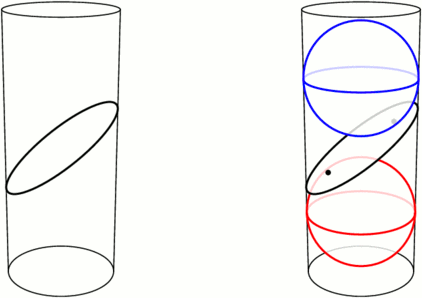Left: An ellipse is formed by slicing a cylinder. Right: Fitting spheres above and below the cut locates the ellipse’s foci.

Take a sphere that snugly fits inside the tube, and drop it down until it touches the ellipse-slice at a single point F1. Do the same with a sphere underneath, touching the slice at F2. These points turn out to be the foci of the ellipse. Let’s see why.

We can use this tubular setup to answer one mystery from earlier: for any point X on the ellipse, the sum of distances of XF1 and XF2 is always the same! The proof lies in the following animation. Segments XF1 and XA have the same length because they’re both tangent to the upper sphere from X, and similarly, XF2=XB. So the sum XF1+XF2 is just the length of segment AB, the height between the spheres’ equators.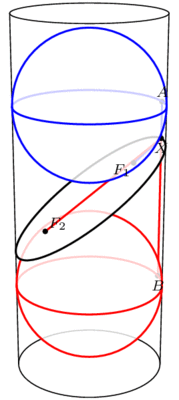The sum F1X+XF2 equals the length of AB and therefore does not change when X moves.

This has two neat consequences. First, it provides an elementary method for drawing ellipses (in real life!): all you need are two push pins and a loop of string, as illustrated below. The string ensures that the sum XF1+XF2 stays fixed while you trace the pen around, as long as you’re careful to keep the string taut throughout.How to draw an ellipse with push-pins and string

Second, what happens if we slice a cone instead of a cylinder? Perhaps surprisingly, we still get an ellipse! Indeed, as above, we can create a sphere on either side of the slice that snugly fits against the slice and the walls of the cone (the so-called Dandelin spheres), and exactly the same proof shows that XF1+XF2 stays constant as X moves around the edge.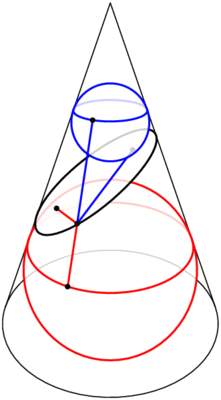Slicing a cone also produces an ellipse, by the same argument.

But wait, there’s still an unanswered question! We’ve seen that the path F1X+XF2 has a fixed length, but why does light bounce off an ellipse along such a path? This is what we really cared about for mini-golf! Come back next time for the answer, and in the meantime, have a great 2 weeks.

# More Putting Predicaments

Last time we discussed some rather challenging holes of (mathematical) mini-golf, uncovering Tokarski’s construction of some un-hole-in-one-able holes. By contrast, here is the all-time easiest hole of mini-golf, namely, a guaranteed hole-in-one: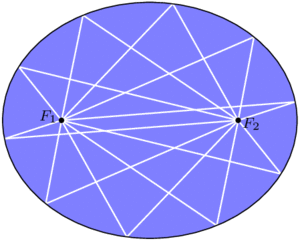In an ellipse, any golf shot from focus $$F_1$$ will bounce directly to the other focus, $$F_2$$.

By some miracle of geometry, if we take an ellipse (i.e., a stretched or squashed circle) and place the ball and cup at two special points $$F_1$$ and $$F_2$$ called the foci of the ellipse, then any golf shot leaving $$F_1$$ will bounce off a wall and proceed directly to $$F_2$$. You can’t miss!

Curiously, this easiest golf hole can be used to construct some even more challenging ones. For starters, consider a mushroom-shaped room as illustrated below (left), where the “mushroom head” is half of an ellipse with foci $$F_1$$ and $$F_2$$. Then the same reflection principle mentioned above tells us that any shot entering the mushroom head via segment $$F_1F_2$$ will be reflected straight back through $$F_1F_2$$ and sent back down the stem. This means that no shot from P can ever reach the triangular “fang” containing Q, and in particular, no hole-in-one from P to Q is possible. In the mirrored-room setting (described last time), if a light source is placed at P, then the whole triangular fang remains unilluminated! This is stronger than Tokarski’s room, where only a single point remained unlit. Similar constructions are possible even with rooms that have no corners, such as the “curvy” mushroom on the right.Left: Any path entering the mushroom head from segment $$F_1F_2$$ will be reflected back down through this segment, so the triangle containing Q is not reachable from P. Right: The same phenomenon is possible even when the room has no corners.

With this idea, we can construct a mirrored room that has dark patches no matter where a light bulb is placed. In the image below, if a light source is placed anywhere in the top half of the room, the lower triangular “fang” will be completely dark. Similarly, the upper fang is not illuminated from anywhere in the bottom half of the room. This room (or one quite like it) was originally designed by Roger Penrose in 1958.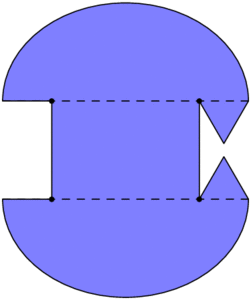This room will have dark regions no matter where the light source is placed.

Back in the mini-golf setting, we can do something even more devious: by chaining multiple Penrose rooms together, we can construct golf holes that require as many shots as we wish! The golf hole below cannot be completed with fewer than 7 shots. Indeed, no single shot can cross two dashed segments with different numbers, so 7 shots are required.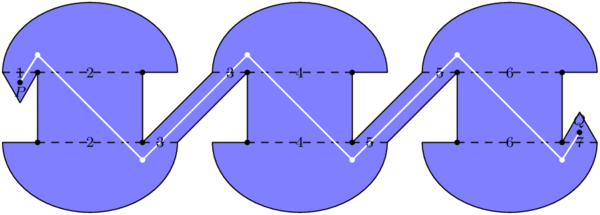At least 7 shots are required to get from P to Q.

### What about polygons?

The golf holes last time were all polygons, whereas we have allowed curved boundaries here. Can we construct a polygonal golf hole, using only flat walls, that still requires at least 7 shots? Or even 3? Unfortunately, the answer this time is “we don’t know, but we think not.” In particular, it has been conjectured that, no matter where the ball and cup are placed in a polygonal golf hole, a single shot can place the ball as close to the hole as desired, so a short second putt can finish the job.

### Notes

1. In fact, even more is true: all of these paths from $$F_1$$ to $$F_2$$ have the same length! We will not prove these facts today. []
2. Assuming you hit hard enough, of course. []
3. For the analysts out there, the room’s boundary can be made smooth. []
4. This too can be accomplished with smooth boundary. []
5. More strongly, O’Rourke and Petrovici conjecture that with a single light source in a polygonal room, the set of unilluminated points has measure 0. Reference: Joseph O’Rourke and Octavia Petrovici. Narrowing Light Rays with Mirrors. Proceedings of the 13th Canadian Conference on Computational Geometry, 137–140, 2001. []